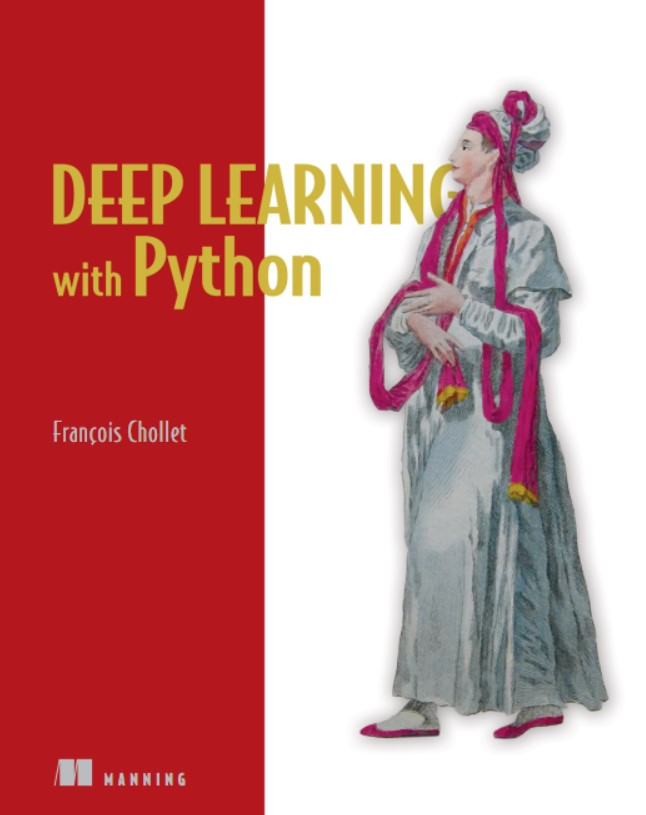# ​DL_WITH_PY系统学习（第3章）1、DL的核心构建
2、Keras的简单介绍
3、搭建DL机器训练环境
4、使用DL模型解决基础问题

3.1 DL的基本构建：layer
layer的定义：以1个或多个tensor作为输入，并且运算出来1个或者多个tensor作为输出的数据处理模型。

from keras import modelsfrom keras import laytersmodel = models.Sequentail()model.add(layers.Dense(32,input_shape=（784，)))model.add(layers.Dense(32))

3.2 DL的基本构建：model
model的定义：一个DL的model是一个直接的、非循环的layer的图，是最直接的layers的线性表示方式，单输入并且但输出。
model包含squence和model两种模式，这在之前已经讨论过。

3.3 DL的基本构建:loss function和optimizers
loss function:它表示的是当前训练项目的成功率；
optimizers:    表示，基于当前的loss function，当前的网络采取什么样的方法优化。主要是体现在SGD的具体变化上。

3.4 DL训练的步骤
3.4.1 定义你的数据集
3.4.2 选择一种layers的组合方式(选择model)
3.4.3 config选择的的model
3.4.4 训练，并且得到结果

a、为什么要使用激活层

b、关于损失函数的选择

loss='binary_crossentropy'

model.compile(optimizer=optimizers.RMSprop(lr=0.001),loss='binary_crossentropy',metrics=['accuracy'])

3.5 fit()返回的是log，它是一个hash，类似这种结构
['acc','loss','val_acc','val_loss']

3.6 predict
model.predict(x_test)

3.7 关于compile中参数的选择，这块的概论非常重要又很模糊

optimizer是什么，loss是什么，metrics是什么。而这3个参数的选择，往往都和问题本身有关：
optimizer:优化器，为预定义优化器名或优化器对象

## 常见的目标函数

rmsprop:绝大多数情况下都是可用的，该优化器通常是面对递归神经网络时的一个良好选择
sgd：（似乎也是可以的）随机梯度下降法，支持动量参数，支持学习衰减率，支持Nesterov动量

loss:categorical:绝对的;cross entropy 交叉熵)
categorical_crossentropy 最好的选择，onehot
sparse_categorical_crossentropy 当你的标签是integer的时候，选择
binary_crossentropy 2类分类的时候，选择
mse mean squared error 当你的问题是回归问题的时候选择(regression）

## 可用的目标函数

• mean_squared_error或mse

• mean_absolute_error或mae

• mean_absolute_percentage_error或mape

• mean_squared_logarithmic_error或msle

• squared_hinge

• hinge

• categorical_hinge

• binary_crossentropy（亦称作对数损失，logloss）

• logcosh

• categorical_crossentropy：亦称作多类的对数损失，注意使用该目标函数时，需要将标签转化为形如(nb_samples, nb_classes)的二值序列

• sparse_categorical_crossentrop：如上，但接受稀疏标签。注意，使用该函数时仍然需要你的标签与输出值的维度相同，你可能需要在标签数据上增加一个维度：np.expand_dims(y,-1)

• kullback_leibler_divergence:从预测值概率分布Q到真值概率分布P的信息增益,用以度量两个分布的差异.

• poisson：即(predictions - targets * log(predictions))的均值

• cosine_proximity：即预测值与真实标签的余弦距离平均值的相反

metrics（列表，包含评估模型在训练和测试时的性能的指标）
acc和accuracy，似乎是一个东西，或者metrics={'ouput_a': 'accuracy'}

3.8 平滑绘制最后的曲线
plt.plot(range(1,len(average_mae_history)+1),average_mae_history)到

def smooth_curve(points,factor=0.9)
smoothed_points = []
for point in points:
if smoothed_points:
previous = smoothed_points[-1]
smoothed_points.append(previous*factor+point*(1-factor))
else:
smothed_points.append(point)
return smoothed_points

posted @ 2018-05-29 09:53 jsxyhelu 阅读(...) 评论(...) 编辑 收藏